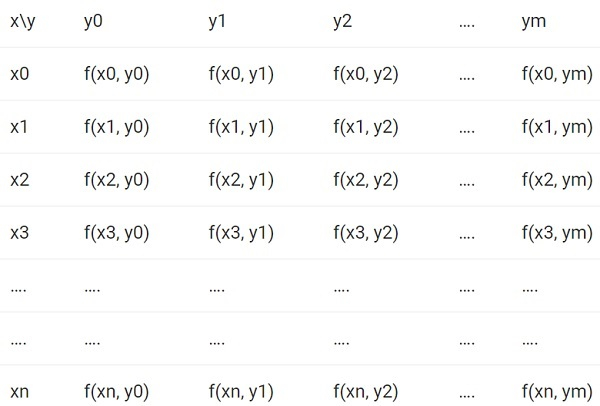# C++ Program to calculate Double Integration

C++Server Side ProgrammingProgramming

We are given with the lower limit of variable x, upper limit of variable x, lower limit of variable y, upper limit of variable y, steps taken for corresponding x and steps taken for corresponding y and the task is to generate the double integration and display the result.

## Example

Input-: steps for x = 1.2
steps for y = 0.54
lower limit of x = 1.3
upper limit of x = 2.1
lower limit of y = 1.0
upper limit for y = 2.1
Output-: double integration is : 2.1

Approach used in the below program is as follows

• Input the value of upper and lower limit of x and y with that input the steps taken for x and y
• The method which we are using for calculating double integration for both x and y is Simpson 1/3 method
• Generate the following table before proceeding further• Apply the simpson 1/3 rule to each row for first integral and repeat it twice for double integration
• Print the result

## ALGORITHM

Start
Step 1-> declare function to  calculate power for integration
float fun(float x, float y)
return pow(pow(x, 4) + pow(y, 5), 0.5)
Step 2-> declare function to find the double integral value
float doubleIntegral(float step_x, float step_y, float lower_x, float upper_x, float lower_y, float upper_y)
Declare int n1, n2
Declare float arr, arr_2, result
set n1 = (upper_x - lower_x) / step_x + 1
set n2 = (upper_y - lower_y) / step_y + 1
Loop For int i = 0 and i < n1 and ++i
Loop For int j = 0 and j < n2 and ++j
set arr[i][j] = fun(lower_x + i * step_x, lower_y + j * step_y)
End
End
Loop For int i = 0 and i < n1 and ++i
set arr_2[i] = 0
Loop For int j = 0 and j < n2 and ++j
IF (j == 0 || j == n2 - 1)
Set arr_2[i] += arr[i][j]
End
Else IF (j % 2 == 0)
Set arr_2[i] += 2 * arr[i][j]
End
Else
set arr_2[i] += 4 * arr[i][j]
End
set arr_2[i] *= (step_y / 3)
End
set result = 0
Loop For int i = 0 and i < n1 and ++i
IF (i == 0 || i == n1 - 1)
set result += arr_2[i]
End
Else IF (i % 2 == 0)
set result += 2 * arr_2[i]
End
Else
set result += 4 * arr_2[i]
End
set result *= (step_x / 3)
End
return result
Step 2-> In main()
declare step for x as float step_x = 1.2
Declare step for y as float step_y = 0.54
Declare lower limit of xfloat lower_x = 1.3
Declare upper limit of xfloat upper_x = 2.1
Declare lower limit of yfloat lower_y = 1.0
Declare upper limit of yfloat upper_y = 2.1
Call (step_x, step_y, lower_x, upper_x, lower_y, upper_y)
Stop

## Example

#include <bits/stdc++.h>
using namespace std;
float fun(float x, float y) {
return pow(pow(x, 4) + pow(y, 5), 0.5);
}
// Function to find the double integral value
float doubleIntegral(float step_x, float step_y, float lower_x, float upper_x, float lower_y, float upper_y) {
int n1, n2;
float arr, arr_2, result;
n1 = (upper_x - lower_x) / step_x + 1;
n2 = (upper_y - lower_y) / step_y + 1;
for (int i = 0; i < n1; ++i) {
for (int j = 0; j < n2; ++j) {
arr[i][j] = fun(lower_x + i * step_x, lower_y + j * step_y);
}
}
for (int i = 0; i < n1; ++i) {
arr_2[i] = 0;
for (int j = 0; j < n2; ++j) {
if (j == 0 || j == n2 - 1)
arr_2[i] += arr[i][j];
else if (j % 2 == 0)
arr_2[i] += 2 * arr[i][j];
else
arr_2[i] += 4 * arr[i][j];
}
arr_2[i] *= (step_y / 3);
}
result = 0;
for (int i = 0; i < n1; ++i) {
if (i == 0 || i == n1 - 1)
result += arr_2[i];
else if (i % 2 == 0)
result += 2 * arr_2[i];
else
result += 4 * arr_2[i];
}
result *= (step_x / 3);
return result;
}
int main() {
float step_x = 1.2; //steps for x
float step_y = 0.54; //steps for y
float lower_x = 1.3; //lower limit of x
float upper_x = 2.1; //upper limit of x
float lower_y = 1.0; //lower limit of y
float upper_y = 2.1; //upper limit of y
cout<<"double integration is : "<<(step_x, step_y, lower_x, upper_x, lower_y, upper_y);
return 0;
}

## Output

double integration is : 2.1